# Hexagon

Draw a regular hexagon inscribed in a circle with radius r=8 cm. What is its perimeter?

Result

p =  48 cm

#### Solution:Leave us a comment of example and its solution (i.e. if it is still somewhat unclear...):

Showing 0 comments:Be the first to comment!#### To solve this example are needed these knowledge from mathematics:

See also our trigonometric triangle calculator.

## Next similar examples:

1. Angle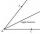Draw angle |∠ ABC| = 130° and built its axis. What angle is between axis angle and arm of angle?
2. TrianglesIvo wants to draw all the triangles whose two sides of which have a length of 4 cm and 9 cm and the length of the third side is expressed in whole centimeters. How many triangles does he have?
3. Triangle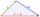Determine if it is possible to construct a triangle with sides 28 31 34 by calculation.
4. Photo frameA square shape photo with a side length of 20 cm is framed by a 4 cm wide bar. Find the external frame size of this photo (the frame is from all sides)
5. Triangle ABCIn a triangle ABC side b measure 10 cm less than the side a and side b is half of the side c. Calculate the length of sides if the circumference of the triangle is 42 cm.
6. Avg speed of flight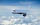The students vice adventure had a 2,367 km flight. If they travel time was 2 hours and 56 minutes, what was their average speed in kilometres per hour?
7. Change in temperatureStarting temperature is 21°C, the highest temperature is 32°C. What is the change in temperature?
8. Speed of carIn 2 hours 40 mins, a car travels 100km. At what speed is the car traveling?
9. Medians 2:1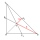Median to side b (tb) in triangle ABC is 12 cm long. a. What is the distance of the center of gravity T from the vertex B? b, Find the distance between T and the side b.
10. Temperature change 2The outside temperature changed -14F over 4 hours. If the temperature changed the same amount each hour, what was the change in temperature each hour?
11. Number of songs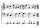Write an expression for the number of songs they need for this show. Evan and Peter have a radio show that has 2 parts. They need 4 fewer than 11 songs in the first part. In the second part, they need 5 fewer than 3 times the number of songs in the first p
12. It is rectangular?Size of two of the angles in a triangle are: α=110°, β=40°. Is it a right triangle?
13. TriangleProve whether you can construct a triangle ABC, if a=9 cm, b=10 cm, c=4 cm.
14. Adding shapes5 triangles + 1 square = how many sides in all
15. PupilsThere are 27 pupils in the classroom. They can swim 21 and ski nine pupils. Neither swim nor ski three pupils. How many pupils can swim and ski?
16. In aboutIn about 12 hours in North Dakota the temperature rose from -33 degrees farenheit to 50 degrees farenheit. By how much did the temperature change?
17. Cyclist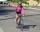Cyclist drives 5 km in half an hour, how long will drive in 3 hours?